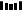# 最小堆的魅力！思路清晰求解「至少需要多少间会议室」### 题目描述

``输入: [[0, 30],[5, 10],[15, 20]]输出: 2``

``输入: [[7,10],[2,4]]输出: 1``

### 参考代码（一）

``public int minMeetingRooms(int[][] intervals) {    if (intervals == null || intervals.length == 0) {        return 0;    }    Arrays.sort(intervals, (int[] a, int[] b) -> (a - b));    PriorityQueue<Integer> pq = new PriorityQueue<>();    pq.offer(intervals);    for (int i = 1; i < intervals.length; ++i) {        if (pq.peek() <= intervals[i]) {            pq.poll();        }        pq.offer(intervals[i]);    }    return pq.size();}``

### 参考代码（二）

``public int minMeetingRooms(int[][] intervals) {    if (intervals == null || intervals.length == 0) {        return 0;    }    int n = intervals.length;    int[] start = new int[n];    int[] end = new int[n];    for (int i = 0; i < n; ++i) {        start[i] = intervals[i];        end[i] = intervals[i];    }    Arrays.sort(start);    Arrays.sort(end);    int s = 0, e = 0;    for (; s < n; s++) {        if (end[e] <= start[s]) {            e++;        }    }    return s - e;}``1.程序员

2.【GitHub

3.【算法动画：七分钟理解什么是KMP算法

4.【数据结构十大经典排序算法动画与解析，看我就够了！▼ 点击『阅读原文』解锁更多图解 LeetCode 题目